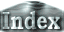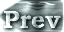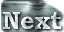# 2. Model and Experimental Design### b. Model

Numerical experiments are performed using the GFD-Dennou-Club AGCM. The basic equations are based on a 3D primitive equation system. The followings are the characteristic features of the model:

• Spatial difference method:
In the horizontal direction, the equations are expanded by spherical harmonics, and are discretized using the pseudospectral method. We use σ as the vertical coordinate, and the equations are discretized in vertical direction according to the method devised by Arakawa and Suarez (1983).
• Cumulus Convection:
Cumulus convection is evaluated using a large-scale condensation and convective adjustment scheme and so-called shallow cumulus convection is not be considered. In the standard cumulus convection parameterization scheme, the approximation that the amount of water vapor is small, q << 1, is used. Since this approximation is inappropriate in the situation considered in this paper, we use a moist convective adjustment scheme which discarded the approximation. (Moist Convective Adjustment Scheme)
• Vertical Turbulent Diffusion:
Turbulent diffusion is modeled using the level-2 closure scheme devised by Mellor and Yamada (1974).
• Ground Surface Flux:
The surface fluxes of sensible heat and latent heat are evaluated with bulk formulas.
• Atmospheric Mass Change Due to Evaporation and Condensation:
A significant increase of water vapor amount is anticipated in the states with an increased solar constant, and since the atmospheric mass will change with evaporation/condensation, the resulting effects are no longer be negligible. Thus, corrections are made to surface pressure using the volume of phase change in the entire atmospheric column. For details, see Change in Surface Pressure by Evaporation and Condensation.
• 2-Grid Noise Suppression:
An increased solar constant produces two-grid noise in the vertical direction that could prevent us from performing long-time integration. To suppress this noise, we introduce damping in the upper seven layers, in addition to the vertical filter for for u, v, T for all layers.
• Horizontal Resolution:
The horizontal resolution will be the minimal resolution required for representing the high- and low-pressures areas, and triangular truncation of total wavenumber 21 (T21) are used.
• Vertical Resolution:
The uppermost vertical grid is located at σ = 10-6 and the total number of levels is 32. See Examination on Vertical Resolution for more information on why this configuration was selected.
• Method of Numerical Calculation:
Time integrations are performed using the backward scheme for the physical process term, the semi-implicit method for the gravity wave term, the Euler scheme for Newtonian cooling, Rayleigh friction, and horizontal diffusion terms. All other terms are integrated using the leapfrog scheme. The time step is basically 20 minutes. However, when the solar constant exceeds 1600 W/m2, 20 minutes is deemed to be too long and is shortened to 10 minutes, and then to 5 minutes as the calculation continues. The Asselin time filter (1972) is used to damp computational modes.

The details of the model referred to in the above section have been compiled in Appendix B for interested readers.

The results for the case where vertical filtering is not implemented are described in Appendix D.